### Learning Objectives

• Explain how the sign of the first derivative affects the shape of a function’s graph.
• State the first derivative test for critical numbers.
• Use concavity and inflection points to explain how the sign of the second derivative affects the shape of a function’s graph.
• Explain the concavity test for a function over an open interval.
• Explain the relationship between a function and its first and second derivatives.
• State the second derivative test for local extrema.

Earlier in this chapter we stated that if a function $f$ has a local extremum at a point $c,$ then $c$ must be a critical number of $f.$ However, a function is not guaranteed to have a local extremum at a critical number. For example, $f(x)={x}^{3}$ has a critical number at $x=0$ since ${f}^{\prime } (x)=3{x}^{2}$ is zero at $x=0,$ but $f$ does not have a local extremum at $x=0.$ Using the results from the previous section, we are now able to determine whether a critical number of a function actually corresponds to a local extreme value. In this section, we also see how the second derivative provides information about the shape of a graph by describing whether the graph of a function curves upward or curves downward.

# The First Derivative Test

Corollary 3 of the Mean Value Theorem showed that if the derivative of a function is positive over an interval $I$ then the function is increasing over $I.$ On the other hand, if the derivative of the function is negative over an interval $I,$ then the function is decreasing over $I$ as shown in the following figure.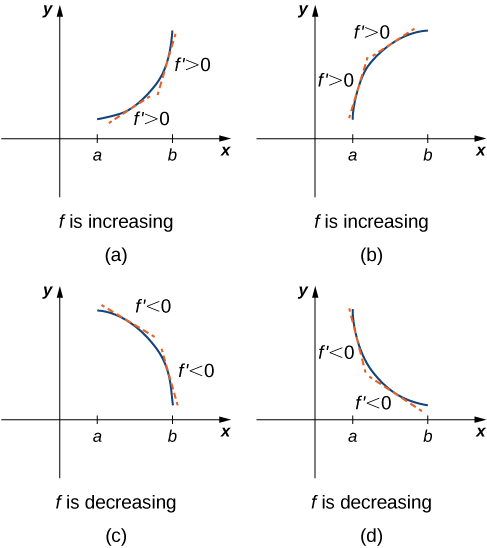A continuous function $f$ has a local maximum at point $c$ if and only if $f$ switches from increasing to decreasing at point $c.$ Similarly, $f$ has a local minimum at $c$ if and only if $f$ switches from decreasing to increasing at $c.$ If $f$ is a continuous function over an interval $I$ containing $c$ and differentiable over $I,$ except possibly at $c,$ the only way $f$ can switch from increasing to decreasing (or vice versa) at point $c$ is if ${f}^{\prime }$ changes sign as $x$ increases through $c.$ If $f$ is differentiable at $c,$ the only way that ${f}^{\prime }.$ can change sign as $x$ increases through $c$ is if ${f}^{\prime }(c)=0.$ Therefore, for a function $f$ that is continuous over an interval $I$ containing $c$ and differentiable over $I,$ except possibly at $c,$ the only way $f$ can switch from increasing to decreasing (or vice versa) is if ${f}^{\prime } (c)=0$ or ${f}^{\prime }(c)$ is undefined. Consequently, to locate local extrema for a function $f,$ we look for points $c$ in the domain of $f$ such that ${f}^{\prime } (c)=0$ or ${f}^{\prime }(c)$ is undefined. Recall that such points are called critical numbers of $f.$

Note that $f$ need not have a local extrema at a critical number. The critical points are candidates for local extrema only. In (Figure) , we show that if a continuous function $f$ has a local extremum, it must occur at a critical number, but a function may not have a local extremum at a critical number. We show that if $f$ has a local extremum at a critical number, then the sign of ${f}^{\prime }$ switches as $x$ increases through that point.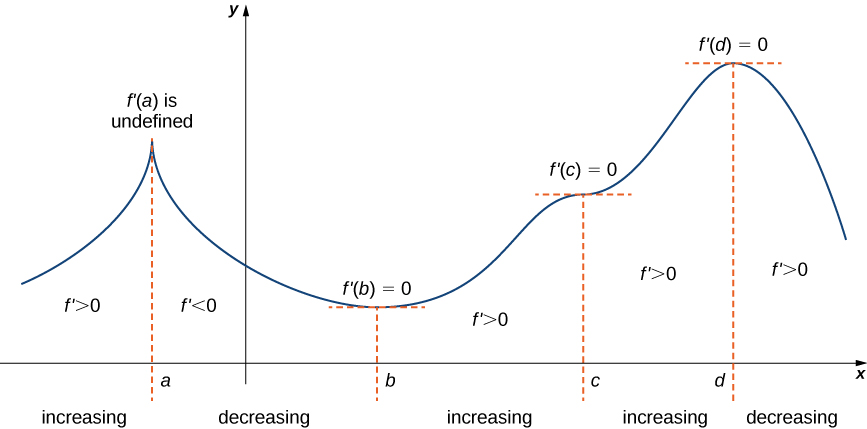Using (Figure) , we summarize the main results regarding local extrema.

• If a continuous function $f$ has a local extremum, it must occur at a critical number $c.$
• The function has a local extremum at the critical number $c$ if and only if the derivative ${f}^{\prime }$ switches sign as $x$ increases through $c.$
• Therefore, to test whether a function has a local extremum at a critical number $c,$ we must determine the sign of ${f}^{\prime }(x)$ to the left and right of $c.$

This result is known as the first derivative test .

### First Derivative Test

Suppose that $f$ is a continuous function over an interval $I$ containing a critical number $c.$ If $f$ is differentiable over $I,$ except possibly at point $c,$ then $f(c)$ satisfies one of the following descriptions:

1. If ${f}^{\prime }$ changes sign from positive when $x < c$ to negative when $x < c,$ then $f(c)$ is a local maximum of $f.$
2. If ${f}^{\prime }$ changes sign from negative when $x < c$ to positive when $x < c,$ then $f(c)$ is a local minimum of $f.$
3. If ${f}^{\prime }$ has the same sign for $x < c$ and $x < c,$ then $f(c)$ is neither a local maximum nor a local minimum of $f.$

We can summarize the first derivative test as a strategy for locating local extrema.

### Problem-Solving Strategy: Using the First Derivative Test

Consider a function $f$ that is continuous over an interval $I.$

1. Find all critical numbers of $f$ and divide the interval $I$ into smaller intervals using the critical numbers as endpoints.
2. Analyze the sign of ${f}^{\prime }$ in each of the subintervals. If ${f}^{\prime }$ is continuous over a given subinterval (which is typically the case), then the sign of ${f}^{\prime }$ in that subinterval does not change and, therefore, can be determined by choosing an arbitrary test point $x$ in that subinterval and by evaluating the sign of ${f}^{\prime }$ at that test point. Use the sign analysis to determine whether $f$ is increasing or decreasing over that interval.
3. Use (Figure) and the results of step 2 to determine whether $f$ has a local maximum, a local minimum, or neither at each of the critical numbers.

Now let’s look at how to use this strategy to locate all local extrema for particular functions.

### Using the First Derivative Test to Find Local Extrema

Use the first derivative test to find the location of all local extrema for $f(x)={x}^{3}-3{x}^{2}-9x-1.$ Use a graphing utility to confirm your results.

#### Solution

Step 1. The derivative is ${f}^{\prime }(x)=3{x}^{2}-6x-9.$ To find the critical numbers, we need to find where ${f}^{\prime }(x)=0.$ Factoring the polynomial, we conclude that the critical numbers must satisfy

$3({x}^{2}-2x-3)=3(x-3)(x+1)=0.$

Therefore, the critical numbers are $x=3,-1.$ Now divide the interval $(-\infty ,\infty )$ into the smaller intervals $(-\infty ,-1),(-1,3)\text{ and }(3,\infty ).$

Step 2. Since ${f}^{\prime }$ is a continuous function, to determine the sign of ${f}^{\prime }(x)$ over each subinterval, it suffices to choose a point over each of the intervals $(-\infty ,-1),(-1,3)\text{ and }(3,\infty )$ and determine the sign of ${f}^{\prime }$ at each of these points. For example, let’s choose $x=-2,x=0,\text{ and }x=4$ as test points.

Interval Test Point Sign of ${f}^{\prime }(x)=3(x-3)(x+1)$ at Test Point Conclusion
$(-\infty ,-1)$ $x=-2$ $(\text{+})(-)(-)=+$ $f$ is increasing.
$(-1,3)$ $x=0$ $(\text{+})(-)(\text{+})=-$ $f$ is decreasing.
$(3,\infty )$ $x=4$ $(\text{+})(\text{+})(\text{+})=+$ $f$ is increasing.

Step 3. Since ${f}^{\prime }$ switches sign from positive to negative as $x$ increases through $1,f$ has a local maximum at $x=-1.$ Since ${f}^{\prime }$ switches sign from negative to positive as $x$ increases through $3,f$ has a local minimum at $x=3.$ These analytical results agree with the following graph.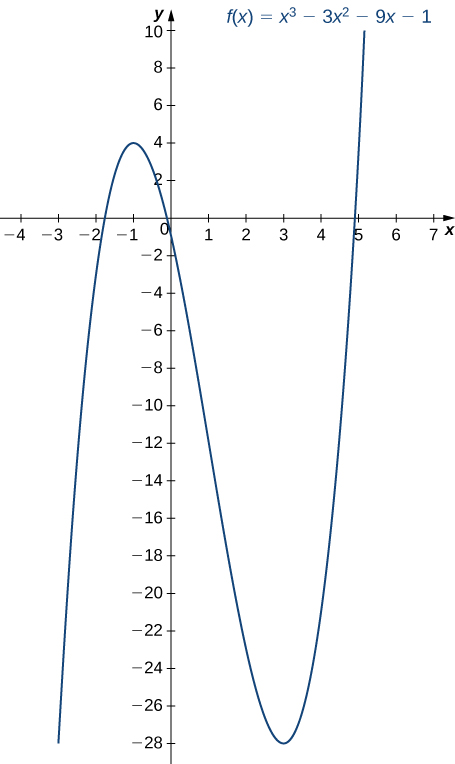Figure 3. The function $f$ has a maximum at $x=-1$ and a minimum at $x=3$

Use the first derivative test to locate all local extrema for $f(x)=-{x}^{3}+\frac{3}{2}{x}^{2}+18x.$

#### Hint

Find all critical numbers of $f$ and determine the signs of ${f}^{\prime }(x)$ over particular intervals determined by the critical numbers.

#### Solution

$f$ has a local minimum at -2 and a local maximum at 3.

### Using the First Derivative Test

Use the first derivative test to find the location of all local extrema for $f(x)=5{x}^{1\text{/}3}-{x}^{5\text{/}3}.$ Use a graphing utility to confirm your results.

#### Solution

Step 1. The derivative is

${f}^{\prime }(x)=\frac{5}{3}{x}^{-2\text{/}3}-\frac{5}{3}{x}^{2\text{/}3}=\frac{5}{3{x}^{2\text{/}3}}-\frac{5{x}^{2\text{/}3}}{3}=\frac{5-5{x}^{4\text{/}3}}{3{x}^{2\text{/}3}}=\frac{5(1-{x}^{4\text{/}3})}{3{x}^{2\text{/}3}}.$

The derivative ${f}^{\prime }(x)=0$ when $1-{x}^{4\text{/}3}=0.$ Therefore, ${f}^{\prime }(x)=0$ at $x=\pm 1.$ The derivative ${f}^{\prime }(x)$ is undefined at $x=0.$ Therefore, we have three critical numbers: $x=0,$ $x=1,$ and $x=-1.$ Consequently, divide the interval $(-\infty ,\infty )$ into the smaller intervals $(-\infty ,-1),(-1,0),(0,1),$ and $(1,\infty ).$

Step 2: Since ${f}^{\prime }$ is continuous over each subinterval, it suffices to choose a test point $x$ in each of the intervals from step 1 and determine the sign of ${f}^{\prime }$ at each of these points. The points $x=-2,x=-\frac{1}{2},x=\frac{1}{2},\text{ and }x=2$ are test points for these intervals.

Interval Test Point Sign of ${f}^{\prime }(x)=\frac{5(1-{x}^{4\text{/}3})}{3{x}^{2\text{/}3}}$ at Test Point Conclusion
$(-\infty ,-1)$ $x=-2$ $\frac{(\text{+})(-)}{+}=-$ $f$ is decreasing.
$(-1,0)$ $x=-\frac{1}{2}$ $\frac{(\text{+})(\text{+})}{+}=+$ $f$ is increasing.
$(0,1)$ $x=\frac{1}{2}$ $\frac{(\text{+})(\text{+})}{+}=+$ $f$ is increasing.
$(1,\infty )$ $x=2$ $\frac{(\text{+})(-)}{+}=-$ $f$ is decreasing.

Step 3: Since $f$ is decreasing over the interval $(-\infty ,-1)$ and increasing over the interval $(-1,0),$ $f$ has a local minimum at $x=-1.$ Since $f$ is increasing over the interval $(-1,0)$ and the interval $(0,1),$ $f$ does not have a local extremum at $x=0.$ Since $f$ is increasing over the interval $(0,1)$ and decreasing over the interval $(1,\infty ),f$ has a local maximum at $x=1.$ The analytical results agree with the following graph.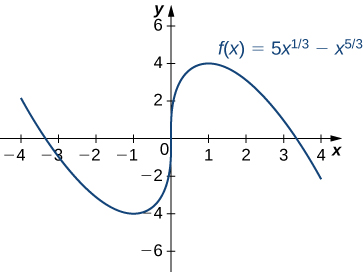Figure 4. The function f has a local minimum at $x=-1$ and a local maximum at $x=1.$

Use the first derivative test to find all local extrema for $f(x)=\sqrt{x-1}.$

#### Hint

The only critical number of $f$ is $x=1.$

#### Solution

$f$ has no local extrema because ${f}^{\prime }$ does not change sign at $x=1.$

# Concavity and Points of Inflection

We now know how to determine where a function is increasing or decreasing. However, there is another issue to consider regarding the shape of the graph of a function. If the graph curves, does it curve upward or curve downward? This notion is called the concavity of the function.

(Figure) (a) shows a function $f$ with a graph that curves upward. As $x$ increases, the slope of the tangent line increases. Thus, since the derivative increases as $x$ increases, ${f}^{\prime }$ is an increasing function. We say this function $f$ is concave up . (Figure) (b) shows a function $f$ that curves downward. As $x$ increases, the slope of the tangent line decreases. Since the derivative decreases as $x$ increases, ${f}^{\prime }$ is a decreasing function. We say this function $f$ is concave down .

### Definition

Let $f$ be a function that is differentiable over an open interval $I.$ If ${f}^{\prime }$ is increasing over $I,$ we say $f$ is concave up over $I.$ If ${f}^{\prime }$ is decreasing over $I,$ we say $f$ is concave down over $I.$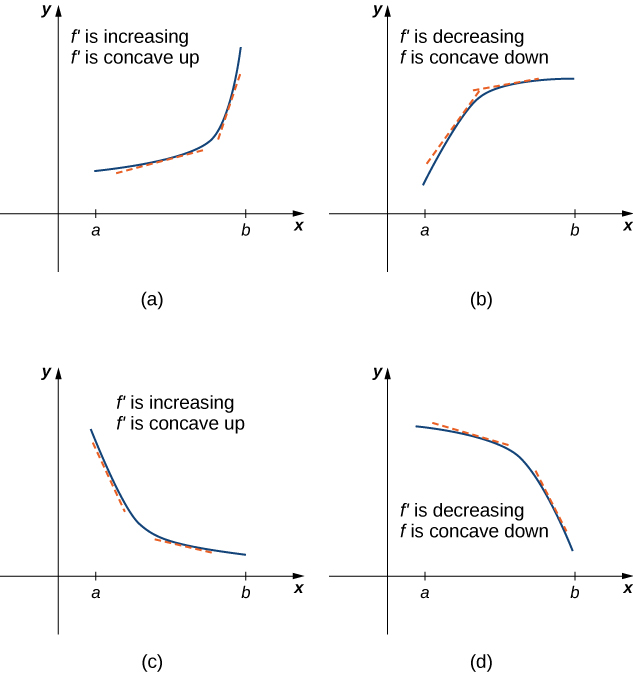Figure 5. (a), (c) Since ${f}^{\prime }$ is increasing over the interval $(a,b),$ we say $f$ is concave up over $(a,b).$ (b), (d) Since ${f}^{\prime }$ is decreasing over the interval $(a,b),$ we say $f$ is concave down over $(a,b).$

In general, without having the graph of a function $f,$ how can we determine its concavity? By definition, a function $f$ is concave up if ${f}^{\prime }$ is increasing. From Corollary 3, we know that if ${f}^{\prime }$ is a differentiable function, then ${f}^{\prime }$ is increasing if its derivative $f''(x) < 0.$ Therefore, a function $f$ that is twice differentiable is concave up when $f''(x) < 0.$ Similarly, a function $f$ is concave down if ${f}^{\prime }$ is decreasing. We know that a differentiable function ${f}^{\prime }$ is decreasing if its derivative $f''(x) < 0.$ Therefore, a twice-differentiable function $f$ is concave down when $f''(x) < 0.$ Applying this logic is known as the concavity test.

### Test for Concavity

Let $f$ be a function that is twice differentiable over an interval $I.$

1. If $f''(x) < 0$ for all $x\in I,$ then $f$ is concave up over $I.$
2. If $f''(x) < 0$ for all $x\in I,$ then $f$ is concave down over $I.$

We conclude that we can determine the concavity of a function $f$ by looking at the second derivative of $f.$ In addition, we observe that a function $f$ can switch concavity ( (Figure) ). However, a continuous function can switch concavity only at a point $x$ if $f''(x)=0$ or $f''(x)$ is undefined. Consequently, to determine the intervals where a function $f$ is concave up and concave down, we look for those values of $x$ where $f''(x)=0$ or $f''(x)$ is undefined. When we have determined these points, we divide the domain of $f$ into smaller intervals and determine the sign of $f''(x)$ over each of these smaller intervals. If $f''(x)$ changes sign as we pass through a point $x,$ then $f$ changes concavity. It is important to remember that a function $f$ may not change concavity at a point $x$ even if $f''(x)=0$ or $f''(x)$ is undefined. If, however, $f$ does change concavity at a point $a$ and $f$ is continuous at $a,$ we say the point $(a,f(a))$ is an inflection point of $f.$

### Definition

If $f$ is continuous at $a$ and $f$ changes concavity at $a,$ the point $(a,f(a))$ is an inflection point of $f.$

< img src="https://s3-us-west-2.amazonaws.com/courses-images/wp-content/uploads/sites/2332/2018/01/11210928/CNX_Calc_Figure_04_05_004.jpg" alt="A sinusoidal function is shown that has been shifted into the first quadrant. The function starts decreasing, so f’ 0. The function reaches the local minimum and starts increasing, so f’ 0 and f’’ 0. It is noted that the slope is increasing for these two intervals. The function then reaches an inflection point (a, f(a)) and from here the slop is decreasing even though the function continues to increase, so f’ 0 and f’’ < 0. The function reaches the maximum and then starts decreasing, so f’
Figure 6. Since $f''(x) < 0$ for $x < a,$ the function $f$ is concave up over the interval $(-\infty ,a).$ Since $f''(x) < 0$ for $x < a,$ the function $f$ is concave down over the interval $(a,\infty ).$ The point $(a,f(a))$ is an inflection point of $f.$

### Testing for Concavity

For the function $f(x)={x}^{3}-6{x}^{2}+9x+30,$ determine all intervals where $f$ is concave up and all intervals where $f$ is concave down. List all inflection points for $f.$ Use a graphing utility to confirm your results.

#### Solution

To determine concavity, we need to find the second derivative $f''(x).$ The first derivative is ${f}^{\prime } (x)=3{x}^{2}-12x+9,$ so the second derivative is $f''(x)=6x-12.$ If the function changes concavity, it occurs either when $f''(x)=0$ or $f''(x)$ is undefined. Since $f''(x)$ is defined for all real numbers $x,$ we need only find where $f''(x)=0.$ Solving the equation $6x-12=0,$ we see that $x=2$ is the only place where $f$ could change concavity. We now test points over the intervals $(-\infty ,2)$ and $(2,\infty )$ to determine the concavity of $f.$ The points $x=0$ and $x=3$ are test points for these intervals.

Interval Test Point Sign of $f''(x)=6x-12$ at Test Point Conclusion
$(-\infty ,2)$ $x=0$ $-$ $f$ is concave down
$(2,\infty )$ $x=3$ $+$ $f$ is concave up.

We conclude that $f$ is concave down over the interval $(-\infty ,2)$ and concave up over the interval $(2,\infty ).$ Since $f$ changes concavity at $x=2,$ the point $(2,f(2))=(2,32)$ is an inflection point. (Figure) confirms the analytical results.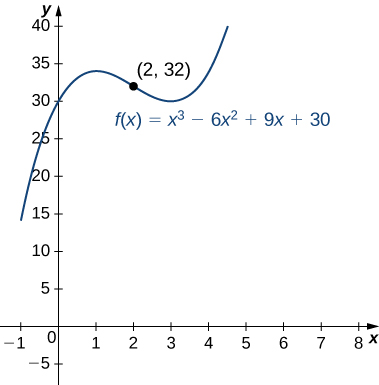Figure 7. The given function has a point of inflection at $(2,32)$ where the graph changes concavity.

For $f(x)=-{x}^{3}+\frac{3}{2}{x}^{2}+18x,$ find all intervals where $f$ is concave up and all intervals where $f$ is concave down.

#### Hint

Find where $f''(x)=0.$

#### Solution

$f$ is concave up over the interval $(-\infty ,\frac{1}{2})$ and concave down over the interval $(\frac{1}{2},\infty )$

We now summarize, in (Figure) , the information that the first and second derivatives of a function $f$ provide about the graph of $f,$ and illustrate this information in (Figure) .

What Derivatives Tell Us about Graphs
Sign of ${f}^{\prime }$ Sign of $f''(x)$ Is $f$ increasing or decreasing? Concavity
Positive Positive Increasing Concave up
Positive Negative Increasing Concave down
Negative Positive Decreasing Concave up
Negative Negative Decreasing Concave down
< img src="https://s3-us-west-2.amazonaws.com/courses-images/wp-content/uploads/sites/2332/2018/01/11210936/CNX_Calc_Figure_04_05_005.jpg" alt="A function is graphed in the first quadrant. It is broken up into four sections, with the breaks coming at the local minimum, inflection point, and local maximum, respectively. The first section is decreasing and concave up; here, f’ 0. The second section is increasing and concave up; here, f’ 0 and f’’ 0. The third section is increasing and concave down; here, f’ 0 and f’’ < 0. The fourth section is increasing and concave down; here, f’
Figure 8. Consider a twice-differentiable function $f$ over an open interval $I.$ If ${f}^{\prime } (x) < 0$ for all $x\in I,$ the function is increasing over $I.$ If ${f}^{\prime } (x) < 0$ for all $x\in I,$ the function is decreasing over $I.$ If $f''(x) < 0$ for all $x\in I,$ the function is concave up. If $f''(x) < 0$ for all $x\in I,$ the function is concave down on $I.$

# The Second Derivative Test

The first derivative test provides an analytical tool for finding local extrema, but the second derivative can also be used to locate extreme values. Using the second derivative can sometimes be a simpler method than using the first derivative.

We know that if a continuous function has a local extrema, it must occur at a critical number. However, a function need not have a local extrema at a critical number. Here we examine how the second derivative test can be used to determine whether a function has a local extremum at a critical number. Let $f$ be a twice-differentiable function such that ${f}^{\prime }(a)=0$ and $f''(x)$ is continuous over an open interval $I$ containing $a.$ Suppose $f''(a) < 0.$ Since $f''(x)$ is continuous over $I,$ $f''(x) < 0$ for all $x\in I$. Then, by Corollary 3, ${f}^{\prime }$ is a decreasing function over $I.$ Since ${f}^{\prime }(a)=0,$ we conclude that for all $x\in I,{f}^{\prime }(x) < 0$ if $x < a$ and ${f}^{\prime }(x) < 0$ if $x < a.$ Therefore, by the first derivative test, $f$ has a local maximum at $x=a.$ On the other hand, suppose there exists a point $b$ such that ${f}^{\prime }(b)=0$ but $f''(x)(b) < 0.$ Since $f''(x)$ is continuous over an open interval $I$ containing $b,$ then $f''(x) < 0$ for all $x\in I$. Then, by Corollary $3,{f}^{\prime }$ is an increasing function over $I.$ Since ${f}^{\prime }(b)=0,$ we conclude that for all $x\in I,$ ${f}^{\prime }(x) < 0$ if $x < b$ and ${f}^{\prime }(x) < 0$ if $x < b.$ Therefore, by the first derivative test, $f$ has a local minimum at $x=b.$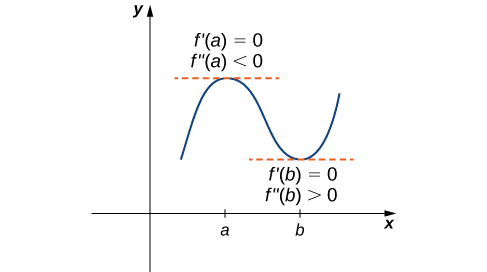Figure 9. Consider a twice-differentiable function $f$ such that $f''(x)$ is continuous. Since ${f}^{\prime } (a)=0$ and $f''(a) < 0,$ there is an interval $I$ containing $a$ such that for all $x$ in $I,$ $f$ is increasing if $x < a$ and $f$ is decreasing if $x < a.$ As a result, $f$ has a local maximum at $x=a.$ Since ${f}^{\prime } (b)=0$ and $f''(x)(b) < 0,$ there is an interval $I$ containing $b$ such that for all $x$ in $I,$ $f$ is decreasing if $x < b$ and $f$ is increasing if $x < b.$ As a result, $f$ has a local minimum at $x=b.$

### Second Derivative Test

Suppose ${f}^{\prime }(c)=0,f''(x)$ is continuous over an interval containing $c.$

1. If $f''(x)(c) < 0,$ then $f$ has a local minimum at $c.$
2. If $f''(x)(c) < 0,$ then $f$ has a local maximum at $c.$
3. If $f''(x)(c)=0,$ then the test is inconclusive.

Note that for case iii. when $f''(x)(c)=0,$ then $f$ may have a local maximum, local minimum, or neither at $c.$ For example, the functions $f(x)={x}^{3},$ $f(x)={x}^{4},$ and $f(x)=-{x}^{4}$ all have critical numbers at $x=0.$ In each case, the second derivative is zero at $x=0.$ However, the function $f(x)={x}^{4}$ has a local minimum at $x=0$ whereas the function $f(x)=-{x}^{4}$ has a local maximum at $x,$ and the function $f(x)={x}^{3}$ does not have a local extremum at $x=0.$

Let’s now look at how to use the second derivative test to determine whether $f$ has a local maximum or local minimum at a critical number $c$ where ${f}^{\prime }(c)=0.$

### Using the Second Derivative Test

Use the second derivative to find the location of all local extrema for $f(x)={x}^{5}-5{x}^{3}.$

#### Solution

To apply the second derivative test, we first need to find critical numbers $c$ where ${f}^{\prime }(c)=0.$ The derivative is ${f}^{\prime }(x)=5{x}^{4}-15{x}^{2}.$ Therefore, ${f}^{\prime }(x)=5{x}^{4}-15{x}^{2}=5{x}^{2}({x}^{2}-3)=0$ when $x=0,\pm \sqrt{3}.$

To determine whether $f$ has a local extrema at any of these points, we need to evaluate the sign of $f''(x)$ at these points. The second derivative is

$f''(x)=20{x}^{3}-30x=10x(2{x}^{2}-3).$

In the following table, we evaluate the second derivative at each of the critical numbers and use the second derivative test to determine whether $f$ has a local maximum or local minimum at any of these points.

$x$ $f''(x)$ Conclusion
$-\sqrt{3}$ $-30\sqrt{3}$ Local maximum
0 0 Second derivative test is inconclusive
$\sqrt{3}$ $30\sqrt{3}$ Local minimum

By the second derivative test, we conclude that $f$ has a local maximum at $x=-\sqrt{3}$ and $f$ has a local minimum at $x=\sqrt{3}.$ The second derivative test is inconclusive at $x=0.$ To determine whether $f$ has a local extrema at $x=0,$ we apply the first derivative test. To evaluate the sign of ${f}^{\prime }(x)=5{x}^{2}({x}^{2}-3)$ for $x\in (-\sqrt{3},0)$ and $x\in (0,\sqrt{3}),$ let $x=-1$ and $x=1$ be the two test points. Since ${f}^{\prime }(-1) < 0$ and ${f}^{\prime }(1) < 0,$ we conclude that $f$ is decreasing on both intervals and, therefore, $f$ does not have a local extrema at $x=0$ as shown in the following graph.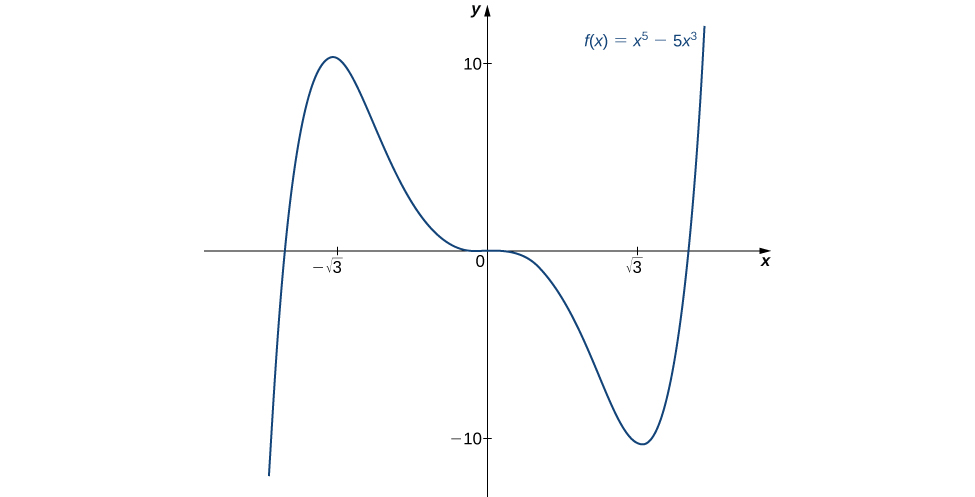Figure 10. The function $f$ has a local maximum at $x=-\sqrt{3}$ and a local minimum at $x=\sqrt{3}$

Consider the function $f(x)={x}^{3}-(\frac{3}{2}){x}^{2}-18x.$ The points $c=3,-2$ satisfy ${f}^{\prime }(c)=0.$ Use the second derivative test to determine whether $f$ has a local maximum or local minimum at those points.

#### Hint

$f''(x)=6x-3$

#### Solution

$f$ has a local maximum at -2 and a local minimum at 3.

We have now developed the tools we need to determine where a function is increasing and decreasing, as well as acquired an understanding of the basic shape of the graph. In the next section we discuss what happens to a function as $x\to \pm \infty .$ At that point, we have enough tools to provide accurate graphs of a large variety of functions.

### Key Concepts

• If $c$ is a critical number of $f$ and ${f}^{\prime }(x) < 0$ for $x < c$ and ${f}^{\prime }(x) < 0$ for $x < c,$ then $f$ has a local maximum at $c.$
• If $c$ is a critical number of $f$ and ${f}^{\prime }(x) < 0$ for $x < c$ and ${f}^{\prime }(x) < 0$ for $x < c,$ then $f$ has a local minimum at $c.$
• If $f''(x) < 0$ over an interval $I,$ then $f$ is concave up over $I.$
• If $f''(x) < 0$ over an interval $I,$ then $f$ is concave down over $I.$
• If ${f}^{\prime }(c)=0$ and $f''(x)(c) < 0,$ then $f$ has a local minimum at $c.$
• If ${f}^{\prime }(c)=0$ and $f''(x)(c) < 0,$ then $f$ has a local maximum at $c.$
• If ${f}^{\prime }(c)=0$ and $f''(x)(c)=0,$ then evaluate ${f}^{\prime }(x)$ at a test point $x$ to the left of $c$ and a test point $x$ to the right of $c,$ to determine whether $f$ has a local extremum at $c.$

1. If $c$ is a critical number of $f(x),$ when is there no local maximum or minimum at $c?$ Explain.

2. For the function $y={x}^{3},$ is $x=0$ both an inflection point and a local maximum/minimum?

#### Solution

It is not a local maximum/minimum because ${f}^{\prime }$ does not change sign

3. For the function $y={x}^{3},$ is $x=0$ an inflection point?

4. Is it possible for a point $c$ to be both an inflection point and a local extrema of a twice differentiable function?

#### Solution

No

5. Why do you need continuity for the first derivative test? Come up with an example.

6. Explain whether a concave-down function has to cross $y=0$ for some value of $x.$

#### Solution

False; for example, $y=\sqrt{x}.$

7. Explain whether a polynomial of degree 2 can have an inflection point.

For the following exercises, analyze the graphs of ${f}^{\prime },$ then list all intervals where $f$ is increasing or decreasing.

8.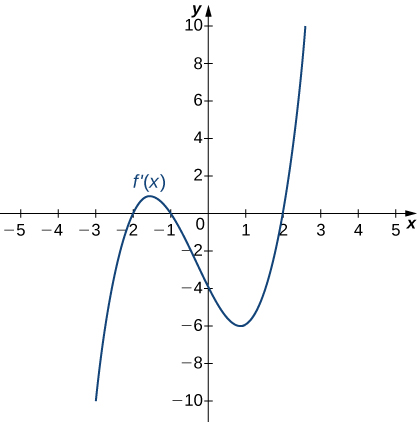#### Solution

Increasing for $-2 < x < -1$ and $x < 2;$ decreasing for $x < -2$ and $-1 < x < 2$

9.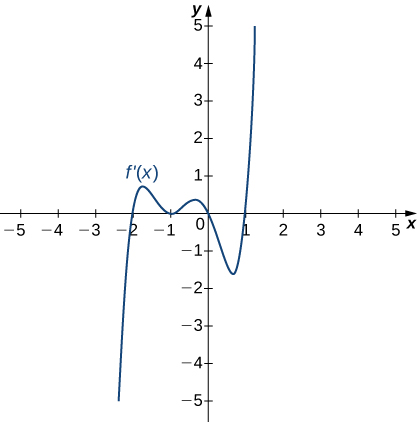10.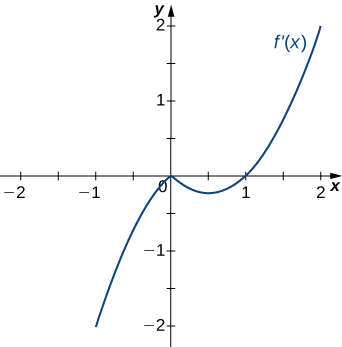#### Solution

Decreasing for $x < 1,$ increasing for $x < 1$

11.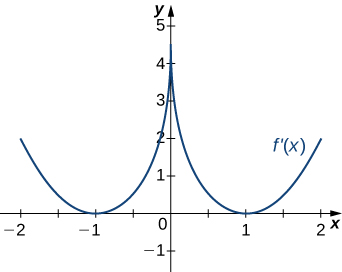12.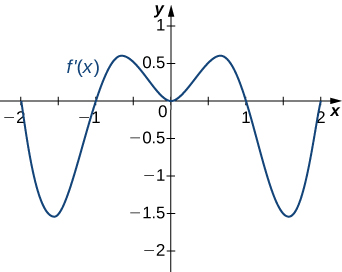#### Solution

Decreasing for $-2 < x < -1$ and $1 < x < 2;$ increasing for $-1 < x < 1$ and $x < -2$ and $x < 2$

For the following exercises, analyze the graphs of ${f}^{\prime },$ then list all intervals where

1. $f$ is increasing and decreasing and
2. the minima and maxima are located.
13.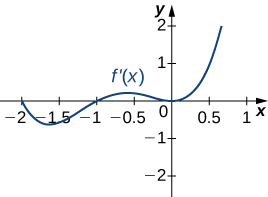14.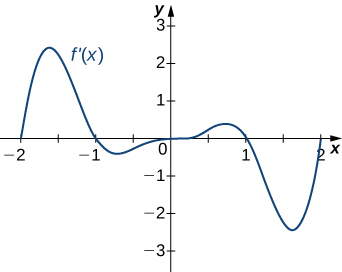#### Solution

a. Increasing over $-2 < x < -1,0 < x < 1,x < 2,$ decreasing over $x < -2,$ $-1 < x < 0,1 < x < 2;$ b. maxima at $x=-1$ and $x=1,$ minima at $x=-2$ and $x=0$ and $x=2$

15.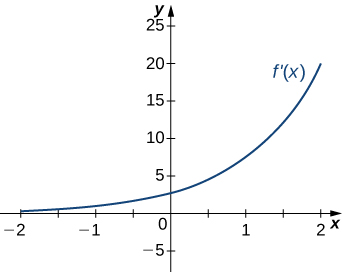16.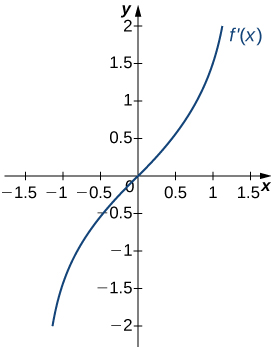#### Solution

a. Increasing over $x < 0,$ decreasing over $x < 0;$ b. Minimum at $x=0$

17.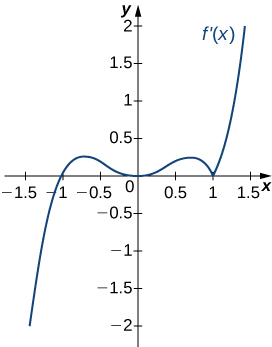For the following exercises, analyze the graphs of ${f}^{\prime },$ then list all inflection points and intervals $f$ that are concave up and concave down.

18.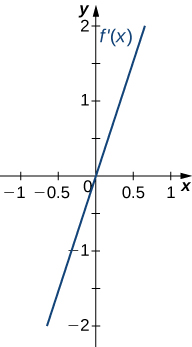#### Solution

Concave up on all $x,$ no inflection points

19.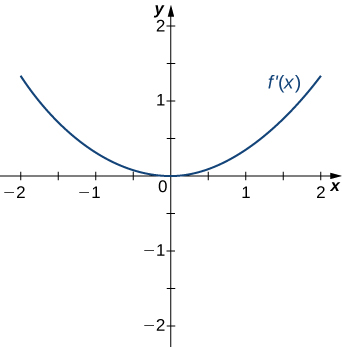20.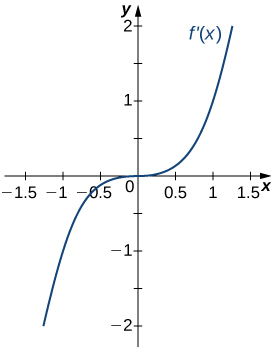#### Solution

Concave up on all $x,$ no inflection points

21.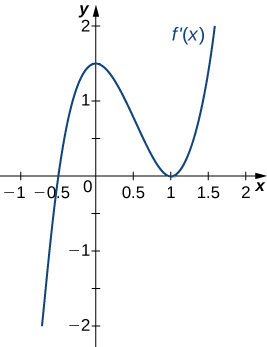22.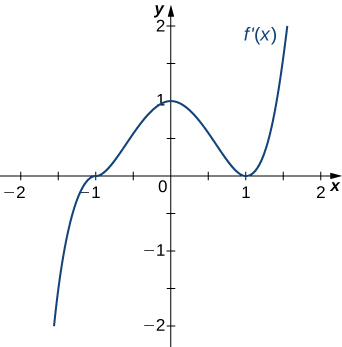#### Solution

Concave up for $x < 0$ and $x < 1,$ concave down for $0 < x < 1,$ inflection points at $x=0$ and $x=1$

For the following exercises, draw a graph that satisfies the given specifications for the domain $x=\left[-3,3\right].$ The function does not have to be continuous or differentiable.

23. $f(x) < 0,{f}^{\prime }(x) < 0$ over $x < 1,-3 < x < 0,{f}^{\prime }(x)=0$ over $0 < x < 1$

24. ${f}^{\prime }(x) < 0$ over $x < 2,-3 < x < -1,{f}^{\prime }(x) < 0$ over $-1 < x < 2,f''(x) < 0$ for all $x$

#### Solution

25. $f''(x) < 0$ over $-1 < x < 1,f''(x) < 0,-3 < x < -1,1 < x < 3,$ local maximum at $x=0,$ local minima at $x=\pm 2$

26. There is a local maximum at $x=2,$ local minimum at $x=1,$ and the graph is neither concave up nor concave down.

#### Solution

27. There are local maxima at $x=\pm 1,$ the function is concave up for all $x,$ and the function remains positive for all $x.$

For the following exercises, determine

1. intervals where $f$ is increasing or decreasing and
2. local minima and maxima of $f.$

28. $f(x)= \sin x+{ \sin }^{3}x$ over $-\pi < x < \pi$

#### Solution

a. Increasing over $-\frac{\pi }{2} < x < \frac{\pi }{2},$ decreasing over $x < -\frac{\pi }{2},x < \frac{\pi }{2}$ b. Local maximum at $x=\frac{\pi }{2};$ local minimum at $x=-\frac{\pi }{2}$

29. $f(x)={x}^{2}+ \cos x$

For the following exercises, determine a. intervals where $f$ is concave up or concave down, and b. the inflection points of $f.$

30. $f(x)={x}^{3}-4{x}^{2}+x+2$

#### Solution

a. Concave up for $x < \frac{4}{3},$ concave down for $x < \frac{4}{3}$ b. Inflection point at $x=\frac{4}{3}$

For the following exercises, determine

1. intervals where $f$ is increasing or decreasing,
2. local minima and maxima of $f,$
3. intervals where $f$ is concave up and concave down, and
4. the inflection points of $f.$

31. $f(x)={x}^{2}-6x$

32. $f(x)={x}^{3}-6{x}^{2}$

#### Solution

a. Increasing over $x < 0$ and $x < 4,$ decreasing over $0 < x < 4$ b. Maximum at $x=0,$ minimum at $x=4$ c. Concave up for $x < 2,$ concave down for $x < 2$ d. Infection point at $x=2$

33. $f(x)={x}^{4}-6{x}^{3}$

34. $f(x)={x}^{11}-6{x}^{10}$

#### Solution

a. Increasing over $x < 0$ and $x < \frac{60}{11},$ decreasing over $0 < x < \frac{60}{11}$ b. Minimum at $x=\frac{60}{11}$ c. Concave down for $x < \frac{54}{11},$ concave up for $x < \frac{54}{11}$ d. Inflection point at $x=\frac{54}{11}$

35. $f(x)=\frac{2x-1}{x^2}$

36. $f(x)={x}^{2}+x+1$

#### Solution

a. Increasing over $x < -\frac{1}{2},$ decreasing over $x < -\frac{1}{2}$ b. Minimum at $x=-\frac{1}{2}$ c. Concave up for all $x$ d. No inflection points

37. $f(x)=2x + \ln|x|$

Given $f, f'$ and $f''$, determine

1. intervals where $f$ is increasing or decreasing,
2. coordinates of local minima and maxima of $f,$
3. intervals where $f$ is concave up and concave down, and
4. coordinates of the inflection points of $f.$

38. $f(x)= \frac{x^2-1}{(x-2)^2}, f'(x) = \frac{2(-2x+1)}{(x-2)^3}, f''(x) = \frac{2(4x+1)}{(x-2)^4}$

#### Solution

a. Increasing over $\left[\frac{1}{2},2)$ decreasing over $(-\infty, \frac{1}{2}]$ and $(2, \infty)$ b. Minimum at $x=\frac{1}{2}$, no local max c. Concave up over $(-\frac{1}{4},2)$ and $(2,\infty)$; Concave down over $(-\infty, -\frac{1}{4})$d. Inflection point at $(-\frac{1}{4}, -\frac{5}{27})$

39. $f(x)= \frac{2x^2}{x^2-1}, f'(x) = \frac{-4x}{(x^2-1)^2}, f''(x) = \frac{4(3x^2+1)}{(x^2-1)^3}$

40. $f(x) = \ln(1+x^2), f'(x) = \frac{2x}{1+x^2}, f''(x) = \frac{2(1-x^2)}{(1+x^2)^2}$

#### Solution

a. Increasing over $(0,\infty)$ decreasing over $(-\infty,0)$ b. Minimum at $(0,0)$, no local max c. Concave up over $(-1,1)$; Concave down over $(-\infty,-1) , \, (1,\infty)$d. Inflection points at $(-1, \ln(2)) , \, (1, \ln(2))$

41. $f(x)= xe^x, f'(x) = e^x(x+1), f''(x) = e^x(x+2)$

For the following exercises, determine

1. intervals where $f$ is increasing or decreasing,
2. local minima and maxima of $f,$
3. intervals where $f$ is concave up and concave down, and
4. the inflection points of $f.$ Sketch the curve, then use a calculator to compare your answer. If you cannot determine the exact answer analytically, use a calculator.

42. [T] $f(x)= \sin (\pi x)- \cos (\pi x)$ over $x=\left[-1,1\right]$

#### Solution

a. Increases over $-\frac{1}{4} < x < \frac{3}{4},$ decreases over $x < \frac{3}{4}$ and $x < -\frac{1}{4}$ b. Minimum at $x=-\frac{1}{4},$ maximum at $x=\frac{3}{4}$ c. Concave up for $-\frac{3}{4} < x < \frac{1}{4},$ concave down for $x < -\frac{3}{4}$ and $x < \frac{1}{4}$ d. Inflection points at $x=-\frac{3}{4},x=\frac{1}{4}$

43. [T] $f(x)=x+ \sin (2x)$ over $x=\left[-\frac{\pi }{2},\frac{\pi }{2}\right]$

44. [T] $f(x)= \sin x+ \tan x$ over $(-\frac{\pi }{2},\frac{\pi }{2})$

#### Solution

a. Increasing for all $x$ b. No local minimum or maximum c. Concave up for $x < 0,$ concave down for $x < 0$ d. Inflection point at $x=0$

45. [T] $f(x)={(x-2)}^{2}{(x-4)}^{2}$

46. [T] $f(x)=\frac{1}{1-x},x\ne 1$

#### Solution

a. Increasing for all $x$ where defined b. No local minima or maxima c. Concave up for $x < 1;$ concave down for $x < 1$ d. No inflection points in domain

47. [T] $f(x)=\frac{ \sin x}{x}$ over $x=\left[-2\pi ,2\pi \right]$ $\left[2\pi ,0)\cup (0,2\pi \right]$

48. $f(x)= \sin (x){e}^{x}$ over $x=\left[-\pi ,\pi \right]$

#### Solution

a. Increasing over $-\frac{\pi }{4} < x < \frac{3\pi }{4},$ decreasing over $x < \frac{3\pi }{4},x < -\frac{\pi }{4}$ b. Minimum at $x=-\frac{\pi }{4},$ maximum at $x=\frac{3\pi }{4}$ c. Concave up for $-\frac{\pi }{2} < x < \frac{\pi }{2},$ concave down for $x < -\frac{\pi }{2},x < \frac{\pi }{2}$ d. Infection points at $x=\pm \frac{\pi }{2}$

49. $f(x)=\text{ln}x\sqrt{x},x < 0$

50. $f(x)=\frac{1}{4}\sqrt{x}+\frac{1}{x},x < 0$

#### Solution

a. Increasing over $x < 4,$ decreasing over $0 < x < 4$ b. Minimum at $x=4$ c. Concave up for $0 < x < 8\sqrt{2},$ concave down for $x < 8\sqrt{2}$ d. Inflection point at $x=8\sqrt{2}$

51. $f(x)=\frac{{e}^{x}}{x},x\ne 0$

For the following exercises, interpret the sentences in terms of $f,{f}^{\prime },\text{ and }f''(x).$

52. The population is growing more slowly. Here $f$ is the population.

#### Solution

$f < 0,{f}^{\prime } < 0,f''(x) < 0$

53. A bike accelerates faster, but a car goes faster. Here $f=$ Bike’s position minus Car’s position.

54. The airplane lands smoothly. Here $f$ is the plane’s altitude.

#### Solution

$f < 0,{f}^{\prime } < 0,f''(x) < 0$

55. Stock prices are at their peak. Here $f$ is the stock price.

56. The economy is picking up speed. Here $f$ is a measure of the economy, such as GDP.

#### Solution

$f < 0,{f}^{\prime } < 0,f''(x) < 0$

For the following exercises, consider a third-degree polynomial $f(x),$ which has the properties ${f}^{\prime }(1)=0,{f}^{\prime }(3)=0.$ Determine whether the following statements are true or false . Justify your answer.

57. $f(x)=0$ for some $1\le x\le 3$

58. $f''(x)=0$ for some $1\le x\le 3$

#### Solution

True, by the Mean Value Theorem

59. There is no absolute maximum at $x=3$

60. If $f(x)$ has three roots, then it has 1 inflection point.

#### Solution

True, examine derivative

61. If $f(x)$ has one inflection point, then it has three real roots.

## Glossary

concave down
if $f$ is differentiable over an interval $I$ and ${f}^{\prime }$ is decreasing over $I,$ then $f$ is concave down over $I$
concave up
if $f$ is differentiable over an interval $I$ and ${f}^{\prime }$ is increasing over $I,$ then $f$ is concave up over $I$
concavity
the upward or downward curve of the graph of a function
concavity test
suppose $f$ is twice differentiable over an interval $I;$ if $f''(x) < 0$ over $I,$ then $f$ is concave up over $I;$ if $f''(x) < 0$ over $I,$ then $f$ is concave down over $I$
first derivative test
let $f$ be a continuous function over an interval $I$ containing a critical number $c$ such that $f$ is differentiable over $I$ except possibly at $c;$ if ${f}^{\prime }$ changes sign from positive to negative as $x$ increases through $c,$ then $f$ has a local maximum at $c;$ if ${f}^{\prime }$ changes sign from negative to positive as $x$ increases through $c,$ then $f$ has a local minimum at $c;$ if ${f}^{\prime }$ does not change sign as $x$ increases through $c,$ then $f$ does not have a local extremum at $c$
inflection point
if $f$ is continuous at $c$ and $f$ changes concavity at $c,$ the point $(c,f(c))$ is an inflection point of $f$
second derivative test
suppose ${f}^{\prime }(c)=0$ and $f''(x)$ is continuous over an interval containing $c;$ if $f''(c) < 0,$ then $f$ has a local minimum at $c;$ if $f''(c) < 0,$ then $f$ has a local maximum at $c;$ if $f''(c)=0,$ then the test is inconclusive